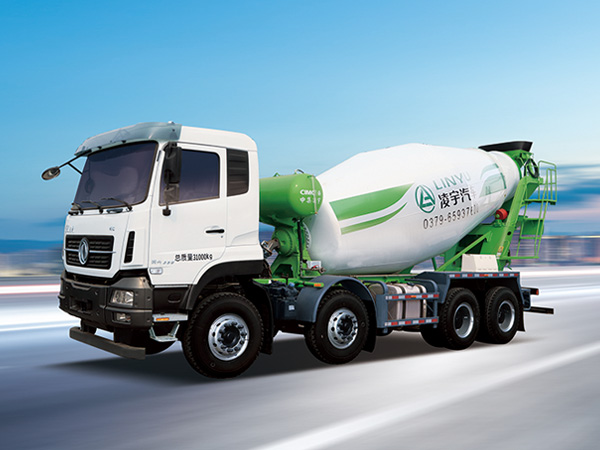# How to calculate the capacity of concrete mixer truck

Concrete mixer truck capacity can vary depending on the manufacturer and model of the truck. However, typical capacities range from 3 to 8 cubic yards, with some larger models having capacities up to 12 cubic yards.

A cubic yard is a unit of volume that is equivalent to 27 cubic feet or 0.76 cubic meters. Therefore, a concrete mixer truck with a capacity of 3 cubic yards can carry up to 81 cubic feet or 2.28 cubic meters of concrete, while a truck with a capacity of 8 cubic yards can carry up to 216 cubic feet or 6.1 cubic meters of concrete.

The capacity of a concrete mixer truck can be calculated by multiplying the truck’s volume by the material density. Here are the steps to calculate the capacity of a concrete mixer truck:Determine the mixer tank volume. This is the volume of the cylindrical mixer tank and is calculated by multiplying the tank radius by the tank height. For example, if the tank radius is 3 feet and the height is 10 feet, then the mixer tank volume is (3 feet)² x 3.14 x 10 feet = 282.6 cubic feet.

Convert the mixer tank volume to cubic yards. Since a cubic yard is equivalent to 27 cubic feet, divide the mixer tank volume by 27 to get the capacity of the mixer in cubic yards. Continuing with the example above, (282.6 cubic feet) / 27 = 10.47 cubic yards.

Determine the concrete density. Concrete density can vary depending on the mix design, but a typical density for normal weight concrete is 150 pounds per cubic foot or 2,400 kg per cubic meter.

For more detailed information about the capacity of concrete mixer trucks, please click to visit:https://www.ly-cimc-linyu.com/a/news/concrete-mixer-truck-capacity.html